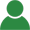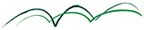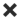ErrorHome Courses Course Calendar Teaching Team About Us Resources Contact
Course Calendar

Correlation and Related Dependency Concepts in Investment Management

Day One

Stylized Facts about Correlations and Dependencies in Financial Market Data

• Contagion effects in stock correlations
• Globalization in global equity investing
• Bonds as a safe haven asset
• Is gold a safe haven?

Workshop: Calculating tail and downside correlations

Mathematical Properties of Correlation and the Correlation Matrix

• Validity of a correlation matrix
• Fixing a broken correlation matrix
• Alternative correlation concepts
• Spearman’s Rank Correlation
• Kendall's T
• Spectral decomposition of a correlation matrix: eigenvalues and eigenvectors
• Singular value decomposition of correlations
• Autocorrelation: dependency over time
• Co-integration and its use in trading

Workshop: Examining the validity of a correlation matrix

Day Two

Scenario Analysis and Stress Testing

• Tweaking individual entries in a correlation matrix
• Changing blocks of correlation values
• Extrapolating trends in correlations: "Risk On" and "Risk Off" scenarios
• Randomizing a correlation matrix
• Handling the additivity of conditional correlations

A General Theory of Dependency – Copulas

• Introduction to Copula Theory
• Applications of Copula Theory
• Data analysis
• Stress testing

Workshop: Identifying copulas in an international asset class universe

Simulating Correlated Data

• Multivariant normal data
• Solutions for non-normal data

Forecasting Correlations

• Historical estimators
• Robust estimators
• Bayesian shrinkage estimators: Jorion, Ledoit/Wolf
• Implied correlations from derivatives instruments
• Deriving asset correlations from factor correlations

Time Series Models for Correlations

• Exponential smoothing
• Multivariate GARCH
• Dynamic Conditional Correlation (DCC)

Workshop: Analyzing the volatility risk of a multi-asset-class portfolio based on robust correlation scenarios